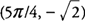## Extreme Value Theorem

An important application of critical points is in determining possible maximum and minimum values of a function on certain intervals. The Extreme Value Theorem guarantees both a maximum and minimum value for a function under certain conditions. It states the following:

• If a function f(x) is continuous on a closed interval [ a, b], then f(x) has both a maximum and minimum value on [ a, b].

The procedure for applying the Extreme Value Theorem is to first establish that the function is continuous on the closed interval. The next step is to determine all critical points in the given interval and evaluate the function at these critical points and at the endpoints of the interval. The largest function value from the previous step is the maximum value, and the smallest function value is the minimum value of the function on the given interval.

Example 1: Find the maximum and minimum values of f(x) = sin x + cos x on [0, 2π].

The function is continuous on [0,2π], and the critcal points areand. The function values at the end points of the interval are f(0) = 1 and f(2π)=1; hence, the maximum function value of f(x) isat x=π/4, and the minimum function value of f(x) is −at x = 5π/4.

Note that for this example the maximum and minimum both occur at critical points of the function.

Example 2: Find the maximum and minimum values of f(x)= x 4−3 x 3−1 on [−2,2].

The function is continuous on [−2,2], and its derivative is f′(x)=4 x 3−9 x 2.Because x=9/4 is not in the interval [−2,2], the only critical point occurs at x = 0 which is (0,−1). The function values at the endpoints of the interval are f(2)=−9 and f(−2)=39; hence, the maximum function value 39 at x = −2, and the minimum function value is −9 at x = 2. Note the importance of the closed interval in determining which values to consider for critical points.